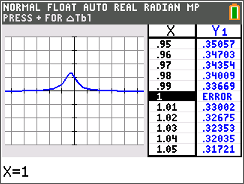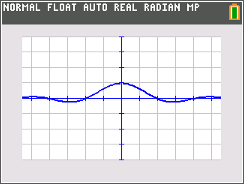••• ##### Device
• TI-84 Plus
• TI-84 Plus Silver Edition
•TI-84 Plus C Silver Edition
•TI-84 Plus CE

# Calculus: Limits of Indeterminant Forms

by Texas Instruments#### Overview

In this activity, students will graph f(x)=sin(x)/x in order to visually determine the limit as x approaches zero. They will confirm the answer numerically by tracing left and right limit points on a graph and looking at values in a table.

#### Key Steps

•In this activity, students begin with an introduction the term indeterminate. Then they graph f (x) = sin(x)/x in order to quickly visually determine the limit as x approaches zero.

•Students set up the table and numerically investigate the values. In order to emphasize that for a limit to exist it must be approaching the same value from both sides, students will examine both sides of zero.